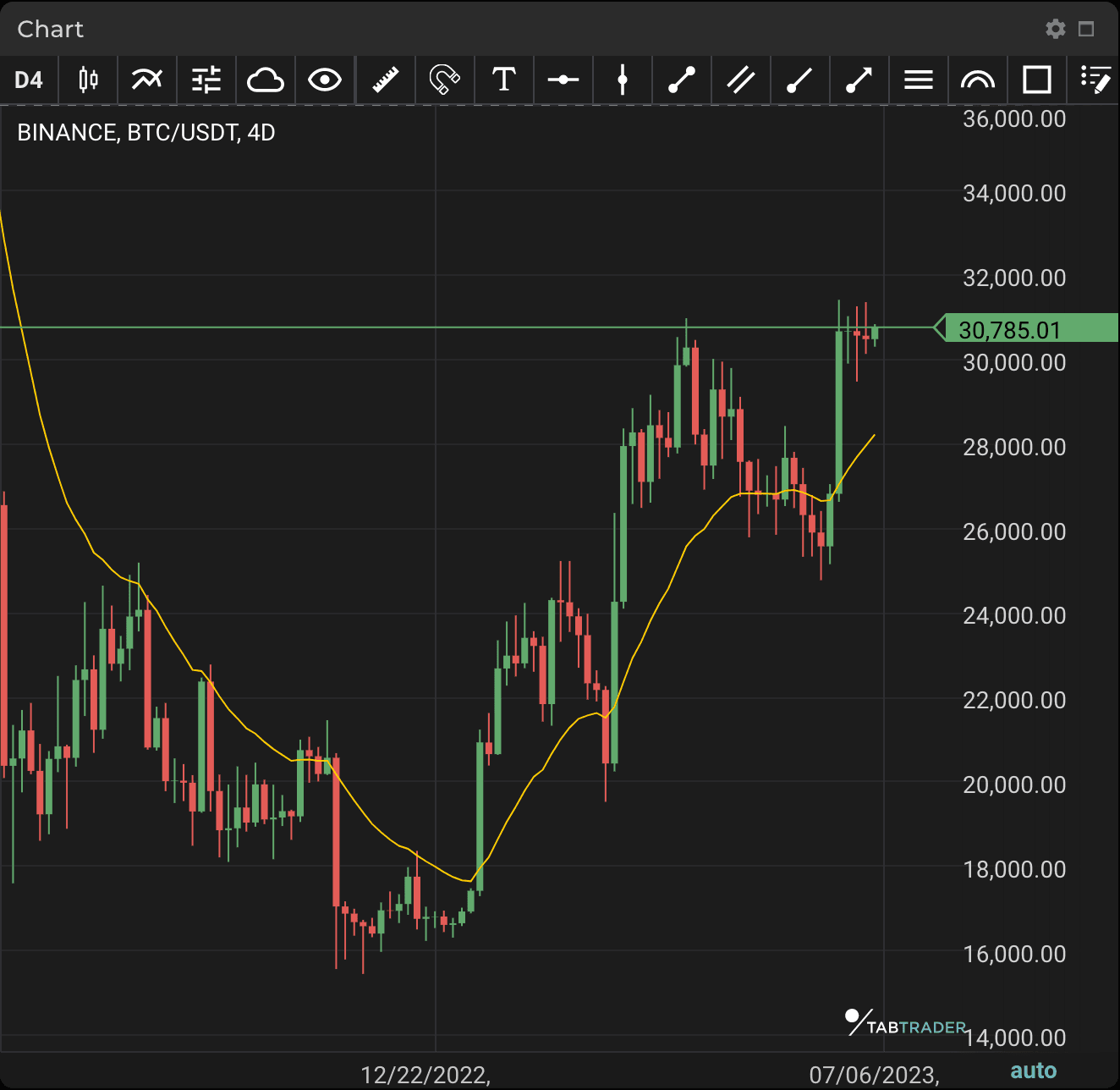# Exponential Moving Average

Publication date is

An Exponential Moving Average (EMA) is a type of moving average (MA) that places greater weight and significance on the most recent data points. The exponential moving average is thus also referred to as the exponentially weighted moving average.

EMA reacts more significantly to recent price changes than a simple moving average (SMA), which applies equal weight to all observations in the period. When price falls to near or just below the EMA, the EMA rises — this is a signal to buy. When price climbs toward or just above the EMA, the EMA falls — this is a sell signal.

Traders often use several different EMA lengths such as 10-period, 50-period and 200-period MAs. EMA may help to trade in the general direction of a trend, but with a delay at entry and exit. Nonetheless, EMA has a shorter delay than SMAs. Moreover, almost all MAs used in technical analysis are lagging indicators. EMA helps to reduce the negative impact of lag because its calculation is based more on the latest data. Note that EMAs are generally used in conjunction with other indicators to confirm significant market moves.EMA

### Calculation

EMA=Price(t)×k+EMA(y)×(1−k)

Where:

t=today

y=yesterday

N=number of periods in EMA

k=2÷(N+1) ​- weighted multiplier

The weighting of the most recent price is greater for a shorter-period EMA compared to a longer-period EMA.

For instance:

Weighted multiplier (10 periods)=2÷(selected time period+1)=2÷(10+1)=0.1818=18.18%

​Weighted multiplier (20 periods)=2÷(selected time period+1)=2÷(20+1)=0.0952=9.52%

Looking to get started in crypto trading?

Try TabTrader on mobile or web!